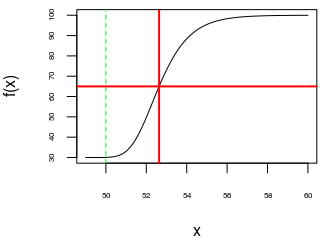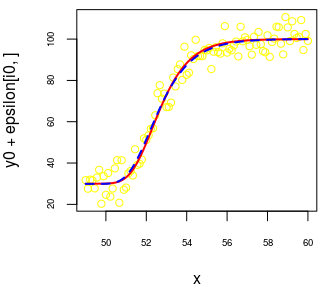Want to share your content on R-bloggers? click here if you have a blog, or here if you don't.

The five-parameters logistic curve is commonly defined by $f(x) = A + \frac{D-A}{\Bigl(1+\exp\bigl(B(C-x)\bigr)\Bigr)^S}.$ Assuming $$B>0$$ and $$S>0$$,

• $$A$$ is the value of the horizontal asymptote when $$x \to -\infty$$;

• $$D$$ is the value of the horizontal asymptote when $$x \to +\infty$$;

• $$B$$ describes how rapidly the curve makes its transition between the two asymptotes;

• $$C$$ is a location parameter, which does not have a nice interpretation (except if $$S=1$$);

• $$S$$ describes the asymmetry of the curve (the curve is symmetric when $$S=1$$).

In the case when $$S=1$$, the parameter $$C$$ is the value of $$x$$ for which the corresponding value $$f(x)$$ is the midpoint between the two asymptotes; moreover, the curve has an inflection point at $$x = C$$.

In the general case, the value of $$x$$ for which the corresponding value $$f(x)$$ is the midpoint between the two asymptotes is $x_{\text{mid}} = C – \frac{\log\Bigl(2^{\frac{1}{S}}-1\Bigr)}{B}.$ It is obtained by solving $$\Bigl(1+\exp\bigl(B(C-x)\bigr)\Bigr)^S = 2$$.

n <- 100
x <- seq(49, 60, length.out = n)
A <- 30; D <- 100; B <- 1; C <- 50; S <- 10
f <- function(x) A + (D-A) / (1 + exp(B*(C-x)))^S
y0 <- f(x)
par(mar = c(4, 4, 0.5, 1))
plot(x, y0, type = "l", cex.axis = 0.5, ylab = "f(x)")
abline(v = C, col = "green", lty = "dashed")
( xmid <- C - log(2^(1/S) - 1)/B )
##  52.63424
abline(v = xmid, col = "red", lwd = 2)
abline(h = (A+D)/2, col = "red", lwd = 2)Note that the inflection point of the curve is not the point correspoding to $$x_{\text{mid}}$$:

library(numDeriv)
par(mar = c(4, 4, 0.5, 1))
plot(x, df, type = "l", cex.axis = 0.5, ylab="f'(x)")
abline(v = xmid, col = "red", lwd = 2)In practice, we are often interested in estimating $$x_{\text{mid}}$$. So it is better to use this other parameterization of the five-parameters logistic curve: $g(x) = A + \frac{D-A}{{\biggl(1+\exp\Bigl(\log\bigl(2^{\frac{1}{S}}-1\bigr) + B(x_{\text{mid}}-x)\Bigr)\biggr)}^S}$ because fitting this curve will directly give the estimate of $$x_{\text{mid}}$$ and its standard error.

Another advantage of this parameterization is that there is a way to get a good starting value of $$x_{\text{mid}}$$ when one wants to fit the five-parameters logistic regression model:

getInitial1 <- function(x, y){
s <- getInitial(y ~ SSfpl(x, A, D, xmid, inverseB),
data = data.frame(x = x, y = y))
c(A = s[["A"]],
B = 1/s[["inverseB"]],
xmid = s[["xmid"]],
D = s[["D"]],
S = 1)
}

I don’t know how to get a good starting value for $$S$$, so I always take $$1$$.

Sometimes, SSfpl can fail. Here is another function which returns some starting values:

getInitial2 <- function(x, y){
NAs <- union(which(is.na(x)), which(is.na(y)))
if(length(NAs)){
x <- x[-NAs]
y <- y[-NAs]
}
low_init <- min(y)
high_init <- max(y)
minmax <- c(which(y == low_init), which(y == high_init))
X <- cbind(1, x[-minmax])
Y <- log((high_init-y[-minmax])/(y[-minmax]-low_init))
fit <- lm.fit(x = X, y = Y)
b_init <- fit$coefficients[] xmid_init <- -fit$coefficients[] / b_init
if(b_init < 0){
b_init <- -b_init
A <- low_init
D <- high_init
}else{
A <- high_init
D <- low_init
}
c(A = A, B = b_init, xmid = xmid_init, D = D, S = 1)
}

Now we wrap these two functions into a single one:

getInitial5PL <- function(x, y){
tryCatch({
getInitial1(x, y)
}, error = function(e){
getInitial2(x, y)
})
}

And finally we can write a function for the fitting:

library(minpack.lm)
fit5pl <- function(x, y){
startingValues <- getInitial5PL(x, y)
fit <- tryCatch({
nlsLM(
y ~ A + (D-A)/(1 + exp(log(2^(1/S)-1) + B*(xmid-x)))^S,
data = data.frame(x = x, y = y),
start = startingValues,
lower = c(-Inf, 0, -Inf, -Inf, 0),
control = nls.lm.control(maxiter = 1024, maxfev=10000))
}, error = function(e){
paste0("Failure of model fitting: ", e\$message)
})
if(class(fit) == "nls" && fit[["convInfo"]][["isConv"]]){
fit
}else if(class(fit) == "nls" && !fit[["convInfo"]][["isConv"]]){
"Convergence not achieved"
}else{ # in this case, 'fit' is the error message
fit
}
}

Let’s try it on a couple of simulated samples:

set.seed(666)
nsims <- 25
epsilon <- matrix(rnorm(nsims*n, 0, 5), nrow = nsims, ncol = n)
estimates <- matrix(NA_real_, nrow = nsims, ncol = 5)
colnames(estimates) <- c("A", "B", "xmid", "D", "S")
for(i in 1:nsims){
fit <- fit5pl(x, y0 + epsilon[i,])
if(class(fit) == "nls"){
estimates[i, ] <- coef(fit)
}else{
estimates[i, ] <- c(NaN, NaN, NaN, NaN, NaN)
}
}
summary(estimates)
##        A               B               xmid             D
##  Min.   :24.19   Min.   :0.8918   Min.   :52.52   Min.   : 98.63
##  1st Qu.:27.99   1st Qu.:0.9566   1st Qu.:52.58   1st Qu.: 99.71
##  Median :29.54   Median :1.0121   Median :52.64   Median :100.31
##  Mean   :29.22   Mean   :1.0367   Mean   :52.63   Mean   :100.22
##  3rd Qu.:30.18   3rd Qu.:1.1207   3rd Qu.:52.67   3rd Qu.:100.64
##  Max.   :32.23   Max.   :1.2599   Max.   :52.76   Max.   :101.80
##        S
##  Min.   :   1.001
##  1st Qu.:   2.262
##  Median :  36.444
##  Mean   :1357.639
##  3rd Qu.:2003.601
##  Max.   :7261.694

The estimate of $$x_{\text{mid}}$$ is excellent. As you can see, the estimate of $$S$$ is sometimes much larger than the true value. Let’s have a look at the worst case:

i0 <- match(max(estimates[, "S"]), estimates[, "S"])
estimates[i0, ]
##            A            B         xmid            D            S
##   29.9159582    0.8917679   52.5992848  100.0519760 7261.6944532
# sample
par(mar = c(4, 4, 0.5, 1))
plot(x, y0 + epsilon[i0, ], col = "yellow", cex.axis = 0.6)
# true curve
curve(A + (D-A)/(1 + exp(log(2^(1/S)-1) + B*(xmid-x)))^S,
add = TRUE, col = "red", lwd = 2)
# fitted curve
with(as.list(estimates[i0, ]),
curve(A + (D-A)/(1 + exp(log(2^(1/S)-1) + B*(xmid-x)))^S,
add = TRUE, col = "blue", lwd = 2, lty = "dashed")
)Thus, while the estimate of $$S$$ is very far from the true value of $$S$$, the fitted curve correctly estimates the true curve. And in such cases, the standard error of the estimate of $$S$$ is big:

fit <- fit5pl(x, y0 + epsilon[i0,])
summary(fit)
##
## Formula: y ~ A + (D - A)/(1 + exp(log(2^(1/S) - 1) + B * (xmid - x)))^S
##
## Parameters:
##       Estimate Std. Error t value Pr(>|t|)
## A    2.992e+01  1.334e+00  22.424   <2e-16 ***
## B    8.918e-01  7.058e-02  12.635   <2e-16 ***
## xmid 5.260e+01  7.310e-02 719.542   <2e-16 ***
## D    1.001e+02  9.347e-01 107.038   <2e-16 ***
## S    7.262e+03  1.757e+06   0.004    0.997
## ---
## Signif. codes:  0 '***' 0.001 '**' 0.01 '*' 0.05 '.' 0.1 ' ' 1
##
## Residual standard error: 5.224 on 95 degrees of freedom
##
## Number of iterations to convergence: 27
## Achieved convergence tolerance: 1.49e-08

Note that nlsLM provides a test of the nullity of $$S$$. This is not interesting, whereas the equality $$S = 1$$ is of interest. So it is better to parametrize the logistic function with $$L = \log(S)$$ instead of $$S$$: $h(x) = A + \frac{D-A}{{\biggl(1+\exp\Bigl(\log\bigl(2^{\exp(-L)}-1\bigr) + B(x_{\text{mid}}-x)\Bigr)\biggr)}^{\exp(L)}}.$ In this way we can get a test of $$L = 0$$, that is $$S = 1$$.